## Table of ContentOpen Access

ARTICLE

# Secrecy Outage Probability for Two-Way Integrated Satellite Unmanned Aerial Vehicle Relay Networks with Hardware Impairments

Xiaoting Ren1, Kefeng Guo2,*

1 College of Public Management, China University of Mining and Technology, Xuzhou, 221116, China
2 School of Space Information, Space Engineering University, Beijing, 101407, China

* Corresponding Author: Kefeng Guo. Email:(This article belongs to this Special Issue: Recent Advances in Backscatter and Intelligent Reflecting Surface Communications for 6G-enabled Internet of Things Networks)

Computer Modeling in Engineering & Sciences 2023, 135(3), 2515-2530. https://doi.org/10.32604/cmes.2023.024857

## Abstract

In this paper, we investigate the secrecy outage performance for the two-way integrated satellite unmanned aerial vehicle relay networks with hardware impairments. Particularly, the closed-form expression for the secrecy outage probability is obtained. Moreover, to get more information on the secrecy outage probability in a high signal-to-noise regime, the asymptotic analysis along with the secrecy diversity order and secrecy coding gain for the secrecy outage probability are also further obtained, which presents a fast method to evaluate the impact of system parameters and hardware impairments on the considered network. Finally, Monte Carlo simulation results are provided to show the efficiency of the theoretical analysis.

## Keywords

1  Introduction

It is reported that satellite communication (SatCom) is considered to be a wishing way for the sixth generation (6G) and beyond next generation (BNG) wireless communication system for its special characters, such as the wide coverage and particular services . Owing to similar reasons, it can make up for the shortage of unmanned aerial vehicle (UAV) networks along with the high data transmission and wide coverage. Based on this foundation, to both utilize the advantage of the SatCom and UAV networks, the framework for the integrated satellite UAV relay network (ISUAVRN) appears, which is considered the major part of the future wireless communication networks [6,7]. Besides, owing to the transmission beam’s wide coverage, many users or relays existed in one beam . In , a selection scheme based on the threshold was provided to keep the balance between the system performance and system complexity for the considered network. Furthermore, the outage probability (OP) was studied. In , one partial selection scheme was utilized to enhance the system performance for the considered networks. In , the authors studied the OP for the cognitive networks, which combined the satellite and the terrestrial networks. In , the OP was researched for the considered networks along with the secondary network selection scheme under the cognitive technology. In , the authors gave a terrestrial and user scheduling scheme based on the maximal performance for the ISUAVRNs in the presence of many terrestrial relays and many users. In , the ergodic capacity for the ISUAVRNs with a selection scheme and multiple terrestrial relays was researched. In , the OP was investigated for the ISUAVRNs with multiple users and an opportunistic user scheduling scheme. To enhance the spectrum efficiency and time utilization, a two-way relay technique is proposed for the ISUAVRNs . In , the OP was researched for the ISUAVRNs with hardware impairments (HIs) and the two-way terrestrial relay. In , the OP was analyzed for the ISUAVRs in the presence of many two-way terrestrial relays and a partial selection scheme. In , the OP was investigated for the considered ISUAVRNs with multiple terrestrial relays and an opportunistic selection scheme under the non-orthogonal multiple access scenario. However, due to the inherent characters of wireless communications particularly for the SatCom, the secrecy issue has been regarded as the major point for the SatCom . The physical layer security (PLS) is considered as the hopeful method to investigate the difference between the eavesdroppers’ and legitimate channels, which is a popular research issue over recent years [19,20]. In , a proposed beamforming (BF) scheme was utilized for the cognitive ISUAVRNs to enhance the secrecy performance. In , the authors researched the secrecy-energy efficient hybrid BF scheme for the ISUAVRNs. In , the non-ideal channel state information (CSI) and cognitive technology were both investigated for the secrecy ISUAVRNs along with secrecy performance. In practical systems, all nodes are not often ideal, which means they always suffer the I/Q imbalance, phase noise, and amplifier non-linearities , which results in the HIs in the transmission nodes. In , all the HIs issues are concluded, which leads to a general HIs model, widely utilized for many former works . Above all, to the authors’ best effort, the investigation for the effects of two-way terrestrial relays on the secrecy ISUAVRNs with HIs is still not published, which motivates our paper.

From the former discussions, by considering the two-way UAV relay and an eavesdropper into our sight, we investigate the secrecy performance for the considered network. The contributions of this paper are presented in the following:

•   By utilizing the two-way UAV relay and an eavesdropper into consideration, the framework for the secrecy ISUAVRN is founded. Besides, the decode-and-forward (DF) mode is used in the UAV to help the source to transmit the signals. Due to the heavy fading and other obstacle reasons, no direct link is assumed for the legitimate transmission link for the two sources. In addition, all the nodes are assumed to suffer from the HIs.

•   Based on the considered system model, the detailed analysis for the secrecy outage probability (SOP) is obtained, which gives the easy method to investigate the SOP. Besides, these theoretical results can direct the engineering guide.

•   To derive the further results of the system parameters on the SOP for the system, the asymptotic behaviors for the SOP are derived, which provide the secrecy diversity and order secrecy coding gain.

The remaining of this paper is shown in the following. A detailed illustration for the considered system is given in Section 2. The analysis for the secrecy OP is derived in Section 3. Some computer simulations namely Monte Carlo (MC) results are given in Section 4 to show the efficiency of the analytical results. The conclusion of the work is provided in Section 5.

Notations: || represents the absolute value of a complex scalar. E[] is the expectation operator, CN(a,B) denotes the complex Gaussian distribution of a random vector a and covariance matrix B. Fy() and fy() represent the cumulative distribution function (CDF) and probability density function (PDF) the of random variable y, respectively. The abbreviations are given in Table 1.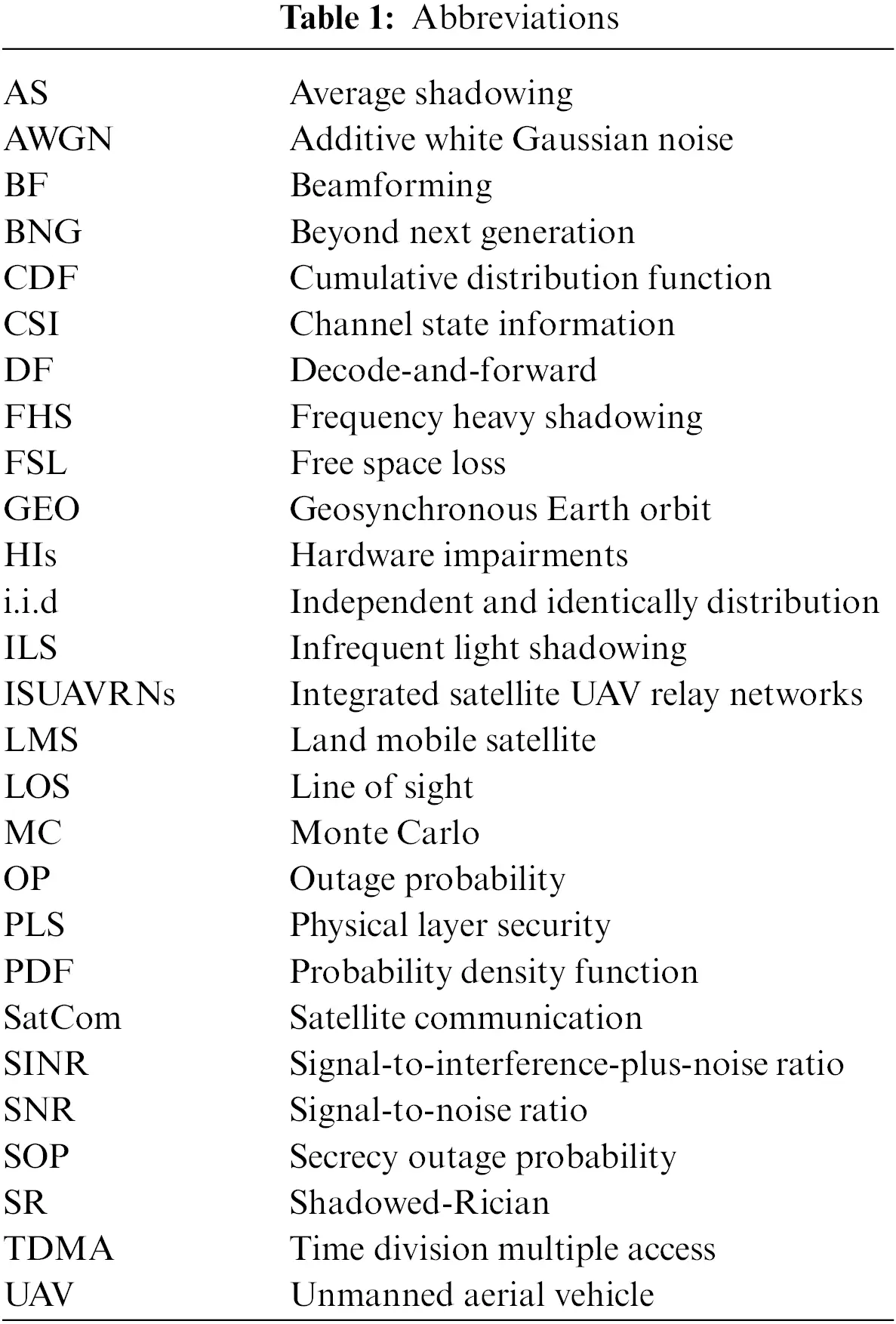2  System Model

As plotted in Fig. 1, we consider an ISUAVRN with HIs in this paper, which contains a satellite source S1, one terrestrial user S2, a two-way UAV R and an eavesdropper E. The two-way UAV R works in DF mode. The whole nodes are considered to have one antenna and suffer from the HIs1. At the same time, an eavesdropper UAV E2 exist in the similar transmission beam with R and wants to overhear the transmission signals from S1, S2 and R. Due to the high building and the other reasons, no direct transmission link is considered between the S1 and S2, which results in that the signal can only be transmitted by R3.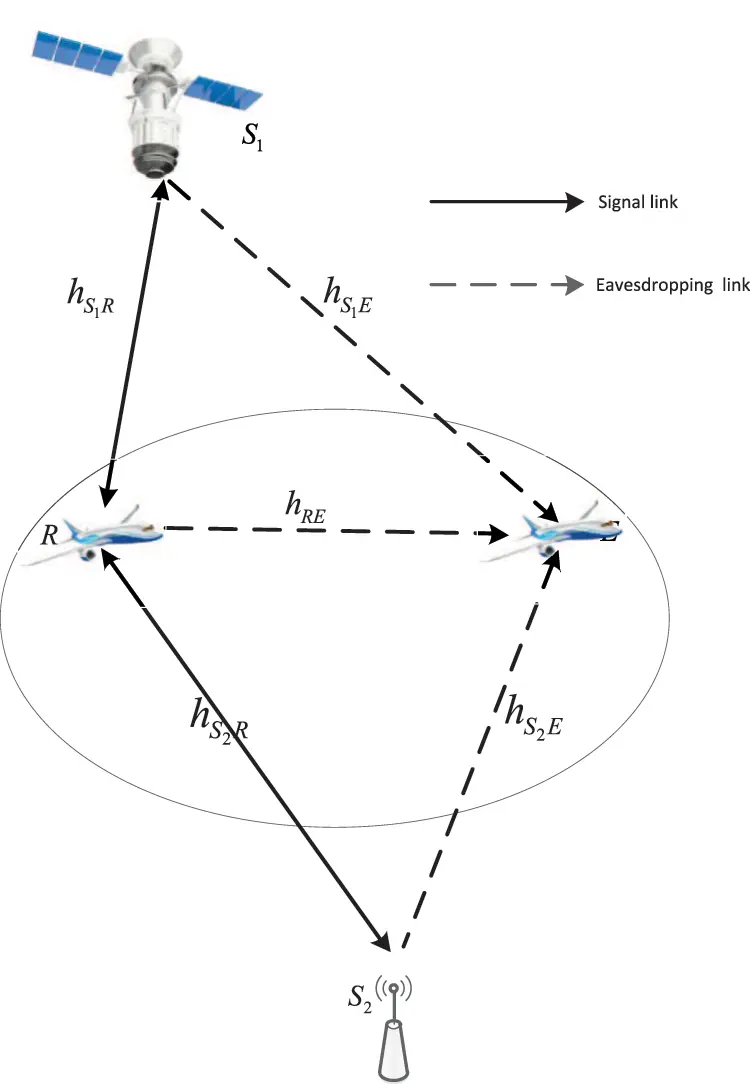Figure 1: The system model description

It will use two time slots for the whole transmission. During the first time slot, S1 and S2 send its own symbols in the same time slot, i.e., s1(t) with E[|s1(t)|2]=1 and s2(t) with E[|s2(t)|2]=1 to R, respectively. So, the obtained signal at R is shown as

yR(t)=PS1hS1R[s1(t)+η1(t)]+PS2hS2R[s2(t)+η2(t)]+nR(t),(1)

where PS1 represents the transmitted power of S1, PS2 represents the power for the S2. hS1R denotes the channel coefficient for the S1R transmission link with modeling as the shadowed-Rician (SR) fading. hS2R represents the channel coefficient for the S2R link with Rayleigh shadowing. As presented before, the transmission nodes suffer from the HIs; η1(t) and η2(t) denote the distortion noise due to HIs at S1 and S2, respectively, which are shown as η1(t)CN(0,k12) and η2(t)CN(0,k22). k1 and k2 represent the HIs level at the S1 and S2, respectively . nR(t) represents the additive white Gaussian noise (AWGN) at R with modeling as nR(t)CN(0,δR2).

Since E and R are located in the same transmission beam, the overhear signal at E in the first time slot is shown as

ySpE(t)=PSphSpE[sp(t)+ηp(t)]+nE(t),p{1,2},(2)

where ySpE(t) represents the signal received at E from the p-th source, PSp denotes the p-th source’s power, hSpE is the channel fading between the p-th source and E with SR and Rayleigh fading, respectively. nE(t) denotes the AWGN at E with modeling as nE(t)CN(0,δE2).

In the second time slot, owing to the utilized DF protocol, the UAV relay will use some techniques to decode the signals received and then re-transmit the re-encoded signal to Sp, respectively. Since Sp knows their own signals, and they can know its signals and delete the self-interference, the obtained signal at Sp is shown as

yRSp(t)=PRhSpR[sp(t)+ηR(t)]+nSp(t),(3)

where PR represents the R’s power, ηR(t) is the distortion noise which comes from the HIs with ηR(t)CN(0,kR2), kR is the impairments’ level at R . nSp(t) denotes the AWGN at Sp which has the presentation as nSp(t)CN(0,δSp2).

As the same assumption, R and E are located in the similar transmission beam. Thus, the obtained signal at E in the second time slot is represented as

yRE(t)=PRhRE[sp(t)+ηR(t)]+nE(t),(4)

where hRE represents the channel coefficient between E and R, which is shown as Rayleigh fading.

By utilizing Eqs. (1) and (3), the obtained signal-to-interference-plus-noise ratio (SINR) at R from the p-th source is obtained as

γS1R=PS1|hS1R|2PS1|hS1R|2k12+PS2|hS2R|2(1+k22)+δR2=γ1S1Rγ1S1Rk12+γ2S2R(1+k22)+1,(5)

γS2R=γ2S2Rγ2S2Rk22+γ1S1R(1+k12)+1,(6)

where γ1S1R=PS1|hS1R|2/δR2 and γ2S2R=PS2|hS2R|2/δR2.

The derived signal-to-noise ratio (SNR) at Sp is given as

γRSp=PR|hRSp|2PR|hRSp|2kR2+δSp2=γRRSpγRRSpkR2+1,(7)

where γRRSp=PR|hRSp|2δSp2.

With the help of Eqs. (2) and (4), the obtained SNR at E is, respectively, obtained as

γSpE=PSp|hSpE|2PSp|hSpE|2kp2+δE2=γpSpEγpSpEkp2+1,(8)

γRE=PR|hRE|2PR|hRE|2kR2+δE2=γRREγRREkR2+1.(9)

where γpSpE=PSp|hSpE|2δE2 and γRRE=PR|hRE|2δE2.

According to , the capacity for secrecy performance has the definition which is shown as the difference between the capacity of the legitimate users’ channel and the eavesdroppers’ channel. By utilizing Eqs. (5)(9), the secrecy capacity for the considered network is represented as

CS=min(CS1,CS2),(10)

where CS1=min(CS11,CS22), CS2=min(CS12,CS21), CS11=[log2(1+γS1R)log2(1+γS1E)]+, CS22=[log2(1+γRS2)log2(1+γRE)]+, CS12=[log2(1+γS2R)log2(1+γS2E)]+ and CS21=[log2(1+γRS1)log2(1+γRE)]+ with [x]+max[x,0].

3  Secrecy System Performance

The detailed analysis for the SOP will be obtained in this part. At first, the channel model for the transmission link is presented.

3.1 The Channel Model

The channel model for the terrestrial transmission link is modeled as independent and identically distribution (i.i.d) Rayleigh fading. From , the PDF and CDF of γX,X{2S2R,RRE,RRS2,2S2E}, are respectively derived as

fγX(x)=1γ¯Xexγ¯X(11)

and

FγX(x)=1exγ¯X,(12)

where γ¯X represents the average channel gain.

The geosynchronous Earth orbit (GEO) satellite is taken for the analysis. In addition, we also consider the satellite having multiple beams for the considered system model. Particularly, time division multiple access (TDMA)  scheme is utilized in the considered model, which means that only one UAV R is suitable to forward the information signal at the next time slot.

The channel coefficient hV,V{1S1R,1S1E,RRS1} between the downlink on-board beam satellite and UAV is presented as

hV=CVfV,(13)

where fV represents the random SR coefficient, CV represents the effects of the antenna pattern and free space loss (FSL), which can be re-given by

CV=λGVGRe4πd2+d02,(14)

where λ denotes the frequency carrier’s wavelength, d is the length between UAV/eavesdropper and the satellite. d035786 km represents the antenna gain for UAV/eavesdropper and GRe represents the satellite’s on-board beam gain.

With help of , GRe can be written as

GRe(dB){G¯max,for0<ϑ<13225logϑ,for1<ϑ<4810,for48<ϑ180,(15)

where G¯max represents the maximum beam gain at the boresight, and ϑ denotes the angle of the off-boresight. Recalling GV, by considering θk as the angle between UAV/eavesdropper position and the satellite. In addition, θ¯k represents the 3 dB angle of satellite beam. The antenna gain can be shown as [2,35]

GVGmax(K1(uk)2uk+36K3(uk)uk3),(16)

where Gmax presents the maximal beam gain, uk=2.07123sinθk/sinθ¯k, K3 and K1 denote the 1st-kind bessel function of order 3 and 1, respectively. In order to gain best performance, thus, θk0 is set, which leads to GVGmax. Relied on this consideration, we derive hV=CVmaxfV with CVmax=λGmaxGRe4πd2+d02.

For fV, a famous SR model was proposed in , which fits land mobile satellite (LMS) communication . By utilizing , the channel coefficient fV can be re-given as fV=f¯V+f~V, where the scattering components f~V follows the i.i.d Rayleigh fading distribution while f¯V represents the element of line of sight (LOS) component which obeys i.i.d Nakagami-m distribution4.

With the help of , the PDF for γV=γ¯VCVmax|fV|2 is given by

fγV(x)=k1=0mV1αV(1mV)k1(δV)k1xk1(k1!)2γ¯Vk1+1exp(ΔVx),(17)

where ΔV=βVδVγ¯V, αV=(2bVmV2bVmV+ΩV)mV/(2bV), βV=1/(2bV) and δV=ΩV2bV(2bVmV+ΩV) with mV0 regarding as the fading severity parameter with integer being. 2bV represents the average power of the multi-path component. ΩV represents the LOS component’s average power. γ¯V represents the transmission link’s average SNR.

Relied on Eq. (17) and utilizing , the CDF of γV is re-derived as

FγV(x)=1k1=0mV1t=0k1αV(1mV)k1(δV)k1xtk1!t!γ¯Vk1+1ΔVk1t+1exp(ΔVx).(18)

3.1.3 Secrecy Outage Probability

From , the SOP defined as

Pout(γ0)=Pr(CSC0)=Pr[min(CS1,CS2)C0]=Pr(CS1C0)+Pr(CS2C0)Pr(CS1C0)Pr(CS2C0),(19)

where C0=log2(1+γ0) with γ0 defined as the target threshold.

Pr(CS1C0)=Pr(CS11C0)+Pr(CS22C0)Pr(CS11C0)Pr(CS22C0),(20)

Pr(CS2C0)=Pr(CS12C0)+Pr(CS21C0)Pr(CS12C0)Pr(CS21C0).(21)

Firstly, with the help of CS11=[log2(1+γS1R)log2(1+γS1E)]+, Pr(CS11C0) can be obtained as

Pr(CS11<C0)=Pr[log2(1+γS1R)log2(1+γS1E)<log2(1+γ0)]=Pr[γS1R<γ0+(γ0+1)γS1E].(22)

From Eq. (22), the first consideration is to obtain the CDF for γS1R and PDF for γS1E. The PDF for γ1S1E has been give in Eq. (17) with V=1S1E, thus utilizing the Eqs. (8) and (17), the PDF for γS1E can be re-written as

fγS1E(x)=1(1k12x)2k11=0mS1E1αS1E(1mS1E)k11(δS1E)k11(k11!)2γ¯S1Ek1+1(x1k12x)k11exp(ΔS1Ex1k12x).(23)

Then by utilizing Eq. (5), the CDF for γS1R is re-written as

FγS1R(x)=Pr(γS1Rx)=Pr(γ1S1Rγ1S1Rk12+γ2S2R(1+k22)+1x)=Pr{γ1S1R[γ2S2R(1+k22)x1k12x+x1k12x]}.(24)

With the help of Eq. (17) with V=1S1R and Eq. (11) with X=2S2R, then we can get

FS1R(x)=1k1=0m1S1R1t=0k1α1S1R(1m1S1R)k1(δ1S1R)k1k1!t!γ¯1S1Rk1+1Δ1S1Rk1t+1γ¯2S2R×0(y(1+k22)x1k12x+x1k12x)texp[Δ1S1R(y(1+k22)x1k12x+x1k12x)yγ¯2S2R]dyI1.(25)

Then, after some mathematic steps, I1 is re-given by

I1=exp(Δ1S1Rx1k12x)p=0t(tp)(x1k12x)t(1+k22)p×0ypexp(Δ1S1R(1+k22)xy1k12xyγ¯2S2R)dyI2.(26)

Next, with the help of , I2 can be obtained as

I2=p!(Δ1S1R(1+k22)x1k12x+1γ¯2S2R)p1.(27)

Then, recalling Eq. (22), Eq. (22) can be re-written as

Pr(CS11<C0)=Pr[γS1R<γ0+(γ0+1)γS1E]=0FγS1R[γ0+(γ0+1)y]fγS1E(y)dy.(28)

Then, it should be mentioned that in Eqs. (23) and (24), y should be satisfied with the following condition, which is y1/k12 and y<1/k12γ0γ0+1. Next, by submitting Eqs. (23) and (24) into Eq. (28), Eq. (28) is rewritten as

Pr(CS11<C0)=0H1FγS1R[γ0+(γ0+1)y]fγS1E(y)dy+H1H1fγS1E(y)dy,(29)

where H1=min(1/k12γ0γ0+1,1k12) and H1=max(1/k12γ0γ0+1,1k12).

However, try the authors’ best efforts, it is too hard to obtain the closed-form expression of Eq. (29), then by utilizing  and utilizing the Gaussian-Chebyshev quadrature , by inserting Eqs. (24) and (23) into Eq. (29), it can be derived as

Pr(CS11<C0)=10H1{1FγS1R[γ0+(γ0+1)y]}fγS1E(y)dy=1k1=0m1S1R1t=0k1k11=0mS1E1p=0t(tp)(1+k22)pp!αS1E(1mS1E)k11(δS1E)k11α1S1R(1m1S1R)k1(δ1S1R)k1(k11!)2γ¯S1Ek1+1k1!t!γ¯1S1Rk1+1Δ1S1Rk1t+1γ¯2S2R×H12l=1N1wlH1(yl),(30)

where

H1(y)=([γ0+(γ0+1)y]1k12[γ0+(γ0+1)y])texp{Δ1S1R[γ0+(γ0+1)y]1k12[γ0+(γ0+1)y]ΔS1Ey1k12y}×(Δ1S1R(1+k22)[γ0+(γ0+1)y]1k12[γ0+(γ0+1)y]+1γ¯2S2R)p1yk11(1k12y)k11+2,

and N1 being the number of the terms, yl=H12(xl+1) denotes the l-th zero of Legendre polynomials, wl represents the Gaussian weight, which can be found in .

By utilizing the similar ways, the closed-form expressions for the Pr(CS22<C0), Pr(CS12<C0), and Pr(CS21<C0) are respectively obtained as

Pr(CS22<C0)=1H22γ¯RREv=1N2ϖvH2(yv),(31)

where

H2(y)=1(1kR2y)2exp{γ0+(γ0+1)yγ¯RRS2{1kR2[γ0+(γ0+1)y]}yγ¯RRE(1kR2y)}

and N2 being the number of the terms, yv=H22(xv+1) represents the v-th zero of Legendre polynomials, ϖv is the Gaussian weight, which is shown in , and H2=min(1/kR2γ0γ0+1,1kR2).

Pr(CS12<C0)=1H32k12=0m1S1R1α1S1R(1m1S1R)k12(δ1S1R)k12k12!γ¯1S1Rk12+1γ¯2S2Rγ¯2S2Eλ¯=1N3ωλ¯H3(yλ¯),(32)

where

H3(y)=1(1k22y)2exp{γ0+(γ0+1)yγ¯2S2R{1γ0+(γ0+1)y}yγ¯2S2E(1k22y)}×{Δ1S1R+(1+k12)[γ0+(γ0+1)y]γ¯2S2R{1k22[γ0+(γ0+1)y]}}k121,

and N3 being the number of the terms, yλ¯=H32(xλ¯+1) represents the λ¯-th zero of Legendre polynomials, ωλ¯ represents the Gaussian weight, which can be seen in , and H3=min(1/k22γ0γ0+1,1k22).

Pr(CS21<C0)=1H42k21=0m1S1R1t=0k12α1S1R(1m1S1R)k12(δ1S1R)k12k12!t!γ¯1S1Rk12+1Δ1S1Rk12t+1γ¯RREμ=1N4ξμH4(yμ),(33)

where

H4(y)=1(1kR2y)2[γ0+(γ0+1)y1kR2γ0kR2(γ0+1)y]texp[Δ1S1Rγ0+Δ1S1R(γ0+1)y1kR2γ0kR2(γ0+1)yyγ¯RRE(1kR2y)]

and N4 being the number of the terms, yμ=H42(xμ+1) represents the μ-th zero of Legendre polynomials, ξμ denotes the Gaussian weight, which has the definition in , and H4=min(1/kR2γ0γ0+1,1kR2).

Then, by substituting Eqs. (30)(33) into Eqs. (19)(21), respectively. The closed-form expression for the SOP will be obtained, which is omitted here.

3.1.4 Asymptotic SOP

In what follows, the asymptotic behaviors for the SOP is obtained. When γ¯L, L{2S2R,1S1R,RRS1,RRS2}, then utilizing exp(x)x01x and (A/x+B)xB, Eqs. (30)(33) will be obtained as

Pr(CS11<C0)=1k1=0m1S1R1t=0k1k11=0mS1E1p=0t(tp)(1+k22)pp!αS1E(1mS1E)k11(δS1E)k11α1S1R(1m1S1R)k1(δ1S1R)k1(k11!)2γ¯S1Ek1+1k1!t!γ¯1S1Rk1+1Δ1S1Rk1t+1γ¯2S2R×H12l=1N1wl([γ0+(γ0+1)yl]1k12[γ0+(γ0+1)yl])t{1Δ1S1R[γ0+(γ0+1)yl]1k12[γ0+(γ0+1)yl]}ylk11(1k12yl)k11+2,(34)

Pr(CS22<C0)=1H22γ¯RREv=1N2ϖv(1kR2yv)2{1γ0+(γ0+1)yvγ¯RRS2{1kR2[γ0+(γ0+1)yv]}},(35)

Pr(CS12<C0)=1H32k12=0m1S1R1α1S1R(1m1S1R)k12(δ1S1R)k12k12!γ¯1S1Rk12+1γ¯2S2Rγ¯2S2Eλ¯=1N3ωλ¯(1k22yλ¯)2×[1γ0+(γ0+1)yλ¯γ¯2S2R[1γ0+(γ0+1)yλ¯]]{Δ1S1R+(1+k12)[γ0+(γ0+1)yλ¯]γ¯2S2R{1k22[γ0+(γ0+1)yλ¯]}}k121,(36)

and

Pr(CS21<C0)=1H42k21=0m1S1R1t=0k12α1S1R(1m1S1R)k12(δ1S1R)k12k12!t!λ¯1S1Rk12+1Δ1S1Rk12t+1γ¯RREμ=1N4ξμ(1kR2yμ)2×[γ0+(γ0+1)yμ1kR2γ0kR2(γ0+1)yμ]t[1Δ1S1Rγ0+Δ1S1R(γ0+1)yμ1kR2γ0kR2(γ0+1)yμ].(37)

Then by substituting Eqs. (34)(37) into Eqs. (19)(21), the asymptotic expression will be derived.

Then from the final asymptotic SOP expression, the secrecy diversity order and secrecy coding gain are respectively derived as

GD=1,(38)

GC=k1=0m1S1R1t=0k1k11=0mS1E1p=0t(tp)(1+k22)pp!αS1E(1mS1E)k11(δS1E)k11α1S1R(1m1S1R)k1(δ1S1R)k1(k11!)2γ¯S1Ek1+1k1!t!(α1S1Rβ1S1R)k1t+1×H12l=1N1wl([γ0+(γ0+1)yl]1k12[γ0+(γ0+1)yl])t{1(α1S1Rβ1S1R)[γ0+(γ0+1)yl]1k12[γ0+(γ0+1)yl]}+H22γ¯RREv=1N2ϖv(1kR2yv)2{1γ0+(γ0+1)yv{1kR2[γ0+(γ0+1)yv]}}+H32k12=0m1S1R1α1S1R(1m1S1R)k12(δ1S1R)k12k12!γ¯2S2E×λ¯=1N3ωλ¯(1k22yλ¯)2[1γ0+(γ0+1)yλ¯[1γ0+(γ0+1)yλ¯]]+H42k21=0m1S1R1t=0k12α1S1R(1m1S1R)k12(δ1S1R)k12k12!t!(α1S1Rβ1S1R)k12t+1γ¯RRE×μ=1N4ξμ(1kR2yμ)2[1(α1S1Rβ1S1R)γ0+(α1S1Rβ1S1R)(γ0+1)yμ1kR2γ0kR2(γ0+1)yμ].(39)

4  Numerical Results

During this part, some representative MC simulations are provided to prove the efficiency of the theoretical analysis. Through these results, the impacts of channel parameters are evaluated. Without loss of any generality, we set γ¯1S1R=γ¯RRS1=γ¯2S2R=γ¯RRS2=γ¯, γ¯1S1E=γ¯2S2E=γ¯RRE=γ¯E and δR2=δS12=δS22=δE2=1, k1=k2=kR=k. The system and channel parameters are shown in Table 2  and Table 3 , respectively.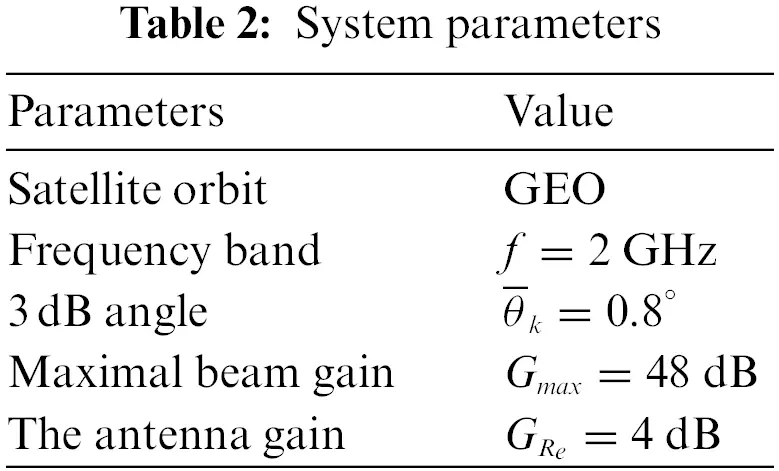Fig. 2 examines the SOP vs. γ¯ for different shadow fading and impairments’ level with γ¯E = 0 dB and γ0 = 0 dB. From Fig. 2, firstly, we can derive that the simulation results are tight across the theoretical analysis, which verify our analysis. In addition, in high SNR regime, the asymptotic results are nearly the same as the simulation results, which show the rightness of the analysis. Moreover, in this figure, it is very interesting that we find the SOP for AS scenario is lower than that of FHS, for the reason that when the channel suffers light fading, the system will have a better system performance. However, we find that the SOP for ILS scenario is the worst, which results in that when the channel is under ILS shadowing, the channel quality for eavesdropper is the best. In ILS scenario, the impact of channel quality on eavesdroppers is superior to that of legitimate users; thus the SOP is the highest. Finally, the lower hardware impairments’ level leads to a lower SOP.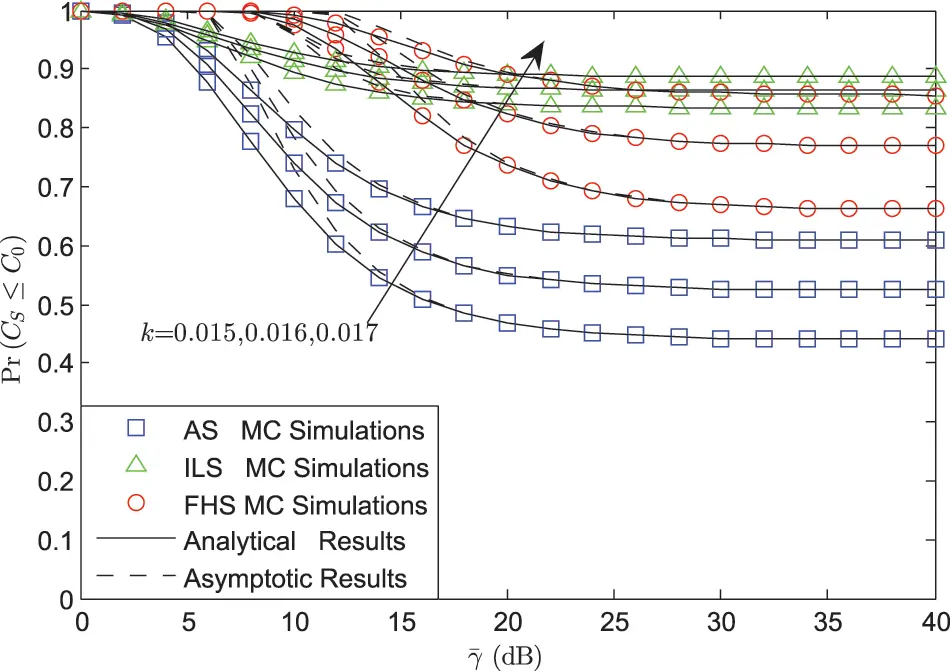Figure 2: SOP vs. γ¯ for different shadow fading and impairments’ level with γ¯E = 0 dB and γ0 = 0 dB

Fig. 3 represents the SOP vs. γ¯ for different γ¯E and impairments’ level under AS scenario. From this figure, we can find that the SOP with lower γ¯E will lead to a lower SOP for the quality of the eavesdroppers gets better. For the reason that a more powerful eavesdropper will derive this phenomenon.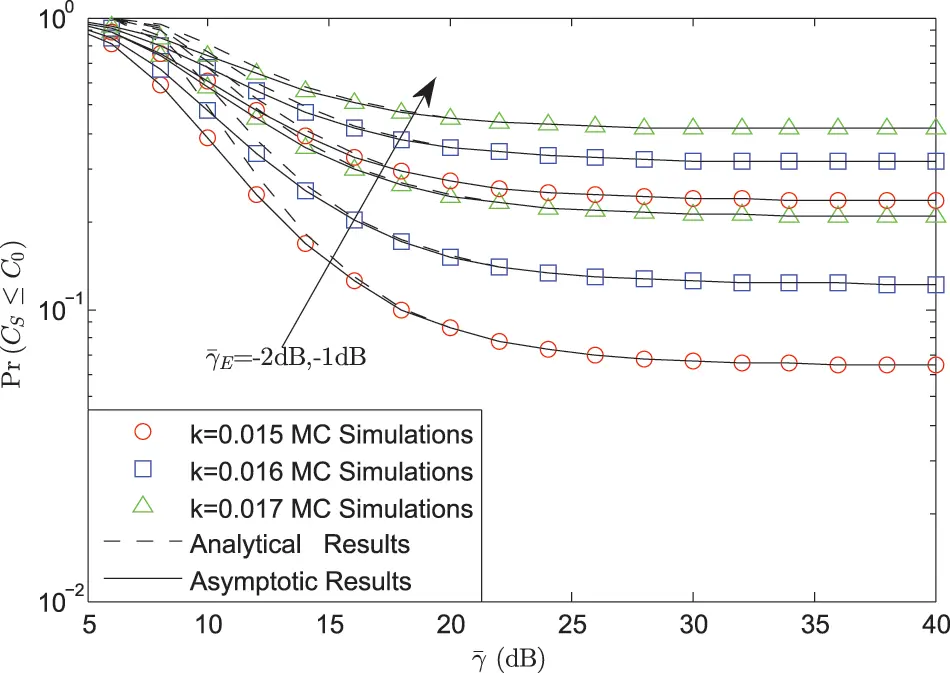Figure 3: SOP vs. γ¯ for different γ¯E and impairments’ level with γ0 = 0 dB under AS scenario

Fig. 4 plots the SOP vs. γ0 for different γ¯E and impairments’ level under AS scenario. It can be derived that, the SOP will be always 1, which means the system is in outage state all the time when the threshold grows to the special value. It can be also seen that this value just has the relationship with the HIs level. In addition, we find that a lower HIs level will bring a larger value. At last, it can be derived that the value for γ¯E has no impact on this value.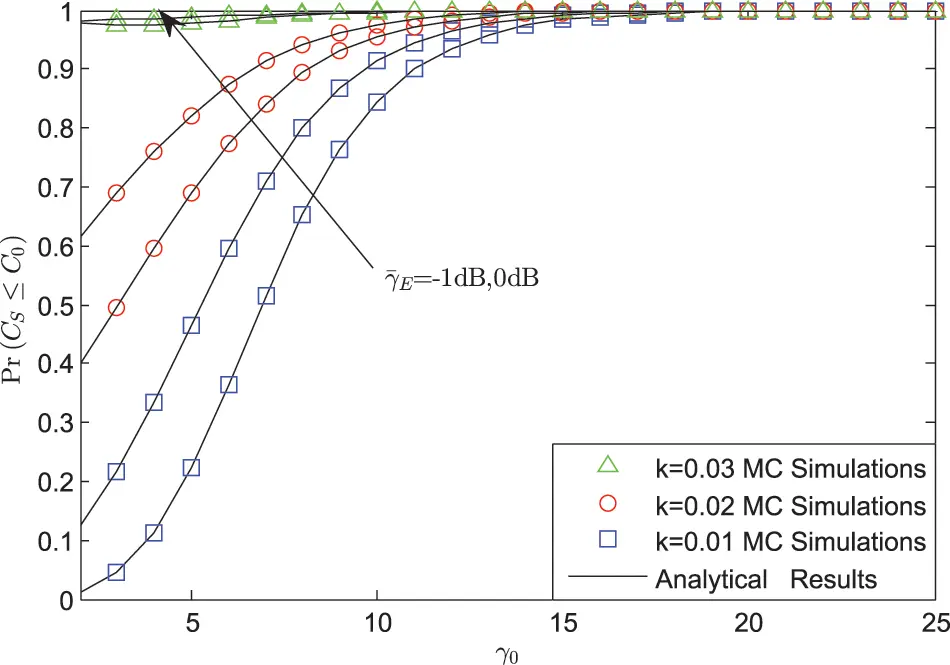Figure 4: SOP vs. γ0 for different γ¯E and impairments’ level under AS scenario

5  Conclusions

This paper investigated the SOP for an integrated satellite UAV relay network with HIs. Especially, the closed-form and asymptotic behaviors for the SOP were derived. Firstly, it was derived that the SOP would have worse performance with threshold being larger; Secondly, it was found that the SOP would be larger with a larger γ¯E; Thirdly, it was seen that the HIs’ level had a great impact on the SOP. A larger impairments’ level brought a larger SOP; Finally, the channel fading also influenced the SOP.

Acknowledgement: The authors wish to express their appreciation to the reviewers for their helpful suggestions which greatly improved the presentation of this paper.

Funding Statement: This work is supported by the Natural Science Foundation of China under Grant No. 62001517.

Conflicts of Interest: The authors declare that they have no conflicts of interest to report regarding the present study.

1We should know that, during this paper, only one antenna is assumed, however the derived results are still fit for the case with many antennas.

2Although this paper just considers one eavesdropper, the derived results are still fit for the model with multiple eavesdroppers. Besides, the model with multiple eavesdroppers will be investigated in our near future.

3Owing to some reasons, direct transmission link between two sources is not available.

4It should be mentioned that, the SR channel is a famous channel model [8,10], which comes from the practical estimation date [37,38].

## References

1. Li, B., Fei, Z., Zhou, C., & Zhang, Y. (2020). Physical layer security in space information networks: A survery. IEEE Internet of Things Journal, 69(5), 33-52. [Google Scholar] [CrossRef]
2. Guo, K., Lin, M., Zhang, B., Zhu, W. P., & Wang, J. B. (2019). On the performance of LMS communication with hardware impairments and interference. IEEE Transactions on Communications, 67(2), 1490-1505. [Google Scholar] [CrossRef]
3. Guo, K., Dong, C., & An, K. (2022). NOMA-Based cognitive satellite terrestrial relay networks: Secrecy performance under channel estimation errors and hardware impairments. IEEE Internet of Things Journal, 9(18), 17334-17347. [Google Scholar] [CrossRef]
4. Liu, R., Guo, K., An, K., & Zhu, S. (2022). NOMA-Based overlay cognitive integrated satellite-terrestrial networks with secondary networks selection. IEEE Transactions on Vehicular Technology, 71(2), 2187-2192. [Google Scholar] [CrossRef]
5. Guo, K., & An, K. (2022). On the performance of RIS-assisted integrated satellite-UAV-terrestrial networks with hardware impairments and interference. IEEE Wireless Communications Letters, 11(1), 131-135. [Google Scholar] [CrossRef]
6. Kodheli, O., Lagunas, E., Maturo, N., Sharma, S. K., & Shankar, B. (2021). Satellite communications in the new space era: A survey and future challenges. IEEE Communications Surveys Tutorials, 23(1), 70-109. [Google Scholar] [CrossRef]
7. Guo, K., Lin, M., Zhang, B., Wang, J. B., & Wu, Y. (2020). Performance analysis of hybrid satellite-terrestrial cooperative networks with relay selection. IEEE Transactions on Vehicular Technology, 69(8), 9053-9067. [Google Scholar] [CrossRef]
8. Guo, K., An, K., Zhang, B., Huang, Y., & Guo, D. (2019). On the performance of the uplink satellite multi-terrestrial relay networks with hardware impairments and interference. IEEE Systems Journal, 13(3), 2297-2308. [Google Scholar] [CrossRef]
9. An, K., Lin, M., Zhu, W. P., Huang, Y., & Zheng, G. (2016). Outage performance of cognitive hybrid satellite-terrestrial networks with interference constraint. IEEE Transactions on Vehicular Technology, 65(11), 9397-9404. [Google Scholar] [CrossRef]
10. Sharma, P. K., Upabhat, P. K., da Costa, D. B., Bithas, P. S., & Kanatas, A. G. (2017). Performance analysis of overlay spectrum sharing in hybrid satellite-terrestrial systems with secondary network selection. IEEE Transactions on Wireless Communications, 16(10), 6586-6601. [Google Scholar] [CrossRef]
11. Upadhyay, P. K., & Sharma, P. K. (2016). Max-max user-relay selection scheme in multiuser and multirelay hybrid satellite-terrestrial relay systems. IEEE Communications Letters, 20(2), 268-271. [Google Scholar] [CrossRef]
12. Zhao, Y., Xie, L., Chen, H., & Wang, K. (2017). Ergodic channel capacity analysis of downlink in the hybrid satellite-terrestrial cooperative system. Wireless Personal Communications, 96(3), 3799-3815. [Google Scholar] [CrossRef]
13. An, K., Lin, M., & Liang, T. (2015). On the performance of multiuser hybrid satellite-terrestrial relay networks with opportunistic scheduling. IEEE Communications Letters, 19(10), 1722-1725. [Google Scholar] [CrossRef]
14. Tang, X., An, K., Guo, K., Wang, S., & Wang, X. (2019). On the performance of two-way multiple relay non-orthogonal multiple access based networks with hardware impairments. IEEE Access, 7, 128896-128909. [Google Scholar] [CrossRef]
15. Guo, K., Zhang, B., Huang, Y., & Guo, D. (2017). Performance analysis of two-way satellite terrestrial relay networks with hardware impairments. IEEE Wireless Communications Letters, 6(4), 430-433. [Google Scholar] [CrossRef]
16. Zeng, W., Zhang, J., Ng, D., Ai, B., & Zhong, Z. (2019). Two-way hybrid terrestrial-satellite relaying systems: Performance analysis and relay selection. IEEE Transactions on Vehicular Technology, 68(7), 7011-7023. [Google Scholar] [CrossRef]
17. Guo, K., An, K., Zhang, B., & Guo, D. (2018). Performance analysis of two-way satellite multi-terrestrial relay networks with hardware impairments. Sensors, 18(5), 1-19. [Google Scholar] [CrossRef]
18. Guo, K., An, K., Zhang, B., Huang, Y., & Tang, X. (2020). Physical layer security for multiuser satellite communication systems with threshold-based scheduling scheme. IEEE Transactions on Vehicular Technology, 69(5), 5129-5141. [Google Scholar] [CrossRef]
19. Li, B., Fei, Z., Chu, Z., Zhou, F., & Wong, K. K. (2018). Robust chance-constrained secure transmission for cognitive satellite-terrestrial networks. IEEE Transactions on Vehicular Technology, 67(5), 4208-4219. [Google Scholar] [CrossRef]
20. Li, B., Fei, Z., Xu, X., & Chu, Z. (2018). Resource allocations for secure cognitive satellite-terrestrial networks. IEEE Wireless Communications Letters, 7(1), 78-81. [Google Scholar] [CrossRef]
21. Lin, Z., Lin, M., Champagne, B., Zhu, W. P., & Naofal, A. D. (2021). Secrecy-energy efficient hybrid beamforming for satellite-terrestrial integrated networks. IEEE Transactions on Communications, 69(9), 6345-6360. [Google Scholar] [CrossRef]
22. An, K., Lin, M., Ouyang, J., & Zhu, W. P. (2016). Secure transmission in cognitive satellite terrstrial networks. IEEE Journal on Selected Areas in Communications, 34(11), 3025-3037. [Google Scholar] [CrossRef]
23. Li, X., Huang, M., Liu, Y., Menon, V. B., & Paul, A. (2021). I/Q imbalance aware nonlinear wireless-powered relaying of B5G networks: Security and reliability analysis. IEEE Transactions Network Science and Engineering, 8(4), 2995-3008. [Google Scholar] [CrossRef]
24. Li, X., Zheng, Y., Alshehri, M. D., Hai, L., Balasubramanian, V. et al. (2021). Cognitive AmBC-NOMA IoV-MTS networks with IQI: Reliability and security analysis. IEEE Transactions Intelligent Transportation Systems. DOI 10.1109/TITS.2021.3113995. [CrossRef]
25. Li, X., Li, J., Liu, Y., Ding, Z., & Nallanathan, A. (2020). Residual transceiver hardware impairments on cooperative NOMA networks. IEEE Transactions on Wireless Communications, 19(1), 680-695. [Google Scholar] [CrossRef]
26. Bjornson, E., Matthaiou, M., & Debbah, M. (2013). A new look at dual-hop relaying: Performance limits with hardware impairments. IEEE Transactions on Communications, 61(11), 4512-4525. [Google Scholar] [CrossRef]
27. Zhang, J., Dai, L., Zhang, X., Bjornson, E., & Wang, Z. (2016). Achievable rate of rician large-scale MIMO channels with transceiver hardware impairments. IEEE Transactions on Vehicular Technology, 65(10), 8800-8806. [Google Scholar] [CrossRef]
28. Budimir, D., Neskovic, N., & Cabarkapa, M. (2016). Hardware impairments impact on fixed-gain AF relaying performance in Nakagami- fading. Electronics Letters, 52(2), 121-122. [Google Scholar] [CrossRef]
29. Duy, T. T., Duong, T. Q., da Costa, D. B., & Bao, V. N. Q. (2015). Proactive relay selection with joint impact of hardware impairments and co-channel interference. IEEE Transactions on Communications, 63(5), 1594-1606. [Google Scholar] [CrossRef]
30. Deng, C., Liu, M., Li, X., & Liu, Y. (2021). Residual transceiver hardware impairments on cooperative NOMA networks. IEEE Systems Journal, 19(1), 680-695. [Google Scholar]
31. Li, X., Zhao, M., Zeng, M., Mumtaz, S., & Menon, V. G. (2021). Hardware impaired ambient backscatter NOMA system: Reliability and security. IEEE Transactions on Communications, 69(4), 2723-2736. [Google Scholar] [CrossRef]
32. Guo, K., Lin, M., Zhang, B., Ouyang, J., & Zhu, W. P. (2018). Secrecy performance of satellite wiretap channels with multi-user opportunistic scheduling. IEEE Wireless Communications Letters, 7(6), 1054-1057. [Google Scholar] [CrossRef]
33. Guo, K., An, K., Zhang, B., Huang, Y., & Guo, D. (2018). Physical layer security for hybrid satellite terrestrial relay networks with joint relay selection and user scheduling. IEEE Access, 6, 55815-55827. [Google Scholar] [CrossRef]
34. Arnau, J., Christopoulos, D., Chatzinotas, S., Mosquera, C., & Ottersten, B. (2014). Performance of the multibeam satellite return link with correlated rain attenuation. IEEE Transactions on Wireless Communications, 13(11), 6286-6299. [Google Scholar] [CrossRef]
35. Guo, K., An, K., Zhou, F., Theodoros, A. T., & Zheng, G. (2021). On the secrecy performance of NOMA-based integrated satellite multipleterrestrial relay networks with hardware impairments. IEEE Transactions on Vehicular Technology, 70(4), 3661-3676. [Google Scholar] [CrossRef]
36. Abdi, A., Lau, W. C., Alouini, M. S., & Kaveh, M. (2003). A new simple model for land mobile satellite channels: First-and second-order statistics. IEEE Transactions on Wireless Communications, 2(3), 519-528. [Google Scholar] [CrossRef]
37. Loo, C. (1985). A statistical model for a land mobile satellite link. IEEE Transactions on Vehicular Technology, 34(3), 122-127. [Google Scholar]
38. Loo, C., & Butterworth, J. S. (1988). Land mobile satellite channel measurements and modeling. Proceeding of the IEEE, 86, 1442-1463. [Google Scholar]
39. Gradshteyn, I. S., Ryzhik, I. M. (2007). Table of integrals, series and products. Boston, USA: Academic Press.
40. Abramowitz, M., Stegun, I. A. (1972). Handbook of mathematical functions with formulas, graphs, and mathematical tables. Florida, USA: CRC Press.

Ren, X., Guo, K. (2023). Secrecy Outage Probability for Two-Way Integrated Satellite Unmanned Aerial Vehicle Relay Networks with Hardware Impairments. CMES-Computer Modeling in Engineering & Sciences, 135(3), 2515–2530.This work is licensed under a Creative Commons Attribution 4.0 International License , which permits unrestricted use, distribution, and reproduction in any medium, provided the original work is properly cited.

View

Like

## Related articles

• Xiaoting Ren, Kefeng Guo
• Ziwei Liu, Xinwei Yue, Shuo Chen,...